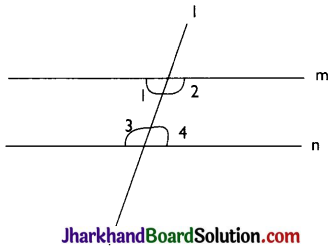# JAC Class 9 Maths Solutions Chapter 5 Introduction to Euclid’s Geometry Ex 5.2

Jharkhand Board JAC Class 9 Maths Solutions Chapter 5 Introduction to Euclid’s Geometry Ex 5.2 Textbook Exercise Questions and Answers.

## JAC Board Class 9th Maths Solutions Chapter 5 Introduction to Euclid’s Geometry Ex 5.2

Page-88

Question 1.
How would you rewrite Euclid’s fifth postulate so that it would be easier to understand?
The fifth postulate is about parallel lines. When two or more lines never intersect each other in a plane and perpendicular distance between them is always constant then they are said to be parallel lines.
Two facts of the postulates:
(i) If P doesn’t lie on 1 then we can draw a line through P which will be parallel to the line 1.
(ii) There will be only one line can be drawn through P which is parallel to the line 1.Question 2.
Does Euclid’s fifth postulate imply the existence of parallel lines? Explain.